# Olympiad Test: Playing With Numbers - 2

## 20 Questions MCQ Test Mathematical Olympiad Class 8 | Olympiad Test: Playing With Numbers - 2

Description
Attempt Olympiad Test: Playing With Numbers - 2 | 20 questions in 40 minutes | Mock test for Class 8 preparation | Free important questions MCQ to study Mathematical Olympiad Class 8 for Class 8 Exam | Download free PDF with solutions
QUESTION: 1

### 763*312, which number should the * be replaced to make the number divisible by 9?

Solution:

For a number to be divisible by 9, its digits sum should be divisible by 9.
∴ 7 + 6 + 3+*+ 3+1+2 = 22 +*.
∴ 5 should be written on place of * to make the number divisible by 9.

QUESTION: 2

### 76215*, the replacement of * by a number gives a number which is divisible by 11, the number will be :

Solution:

⇒ Sum of digits at odd places
= * + 1 + 6 = * +7
Sum of digits at even places
= 5 + 2 + 7
= 14
For divisibility with 11, *+ 7 = 14
⇒ * = 7

QUESTION: 3

###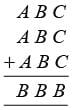, the values of A, B, C are digits from 1 to 9. What will be value of B?

Solution:

3c = x B, where, x is the carry,
∴ x + 3B = y B, where y is the carry.
∴ y + 3A = B.
∴ C = 8, B = 4, A = 1.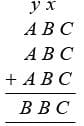QUESTION: 4

What will be the sum of first 22 natural numbers, which, are even?

Solution:

Sum of first 22 even natural numbers
= (2 + 4 + …… + 44)
= 2 (1 + 2 + …… + 22)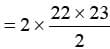= 2 × 11 × 23
= 22 × 23
= 506

QUESTION: 5

One candle was guaranteed to burn for 6 hours, the other for 2 hours. They were both lit at same time. After some time one candle was twice as long as the other. For how long had they been burning?

Solution:

Let the candles have burnt for ‘x’ hours,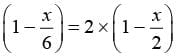⇒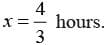QUESTION: 6

Which is a 3-digit numbers, such that all its digits are prime and the 3 digits are the factors of the number?

Solution:

735 is a number having all its digits, a prime number and all the digits of 735 are the factors of 735.

QUESTION: 7

Complete the square given below, and find the value of the sum of missing numbers. The sum of the magic square is 34.​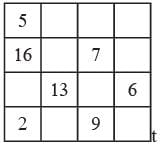Solution: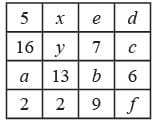We have to find, the value of
(a+b+c+d +e)+(x+ y + z)+ f = x
∴ Sum of numbers of any row/column = 34
∴ a +b+13+6= x+ y + z +13
= e+b +7 +9=c + d +6+ f
= 5 + 16 + a + 2 = x +e+d + 5
= 16 + y + 7 +c= 2 + z + 9 + f = 34
⇒ 2(a+b+c+d +e+ f + x+ y+ z)
+19 + 13 + 16 + 6 + 23+ 5
+23 + 11 34 x 8
⇒ 2x + 116 = 272
⇒ x = 78

QUESTION: 8

Three numbers are such that their sum is 10 and their product is maximum. The product will be :

Solution:

Let the natural numbers be, y and z.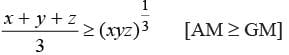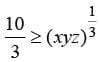⇒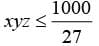⇒ xyz ≤ 37.03
∴ The limiting value of xyz is 37.03,
∴ The numbers x,y and z are natural.
∴ 37.03 cannot be obtained as a product.
∴ 37 is a prime number.
∴ 36 is the greatest number which can be obtained as a product of 3 natural numbers whose sum is 10.

QUESTION: 9

What will be the one’s place digit of 6222?

Solution:

60 = 1
61 = 6
62 = 36
63 = 216
64 = 1296
65 = 7776
∴ It is observed that 6n has 6 at its units place.
∴ 6222 has 6 as its unit’s place  digit.

QUESTION: 10

Find the smallest number which can be expressed as the sum of two cubes of natural numbers.

Solution:

(a) 1729 = (12)3 + (1)3= (10)3 + (1)3
∴ 1729 can be expressed as sum of two perfect natural cubes.
It is the smallest number to satisfy this condition, and, is known as Ramanujan’s Number.

QUESTION: 11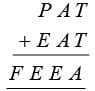, where, P, A, T, E, F are digits from to what will be the value of F?

Solution: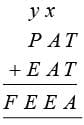x,y are respective carries.
y + P + E + F E
x + 2A = y E
T + T = x A
⇒ P = 9, A = 8, T = 4, E = 6 and F = 1
∴ F = 1

QUESTION: 12

Sum of 3 numbers = product of 3 numbers. If the numbers are consecutive and natural. Find the triplet having least value, of their sum.

Solution:

xyz = x + y + z …(i) We also know that,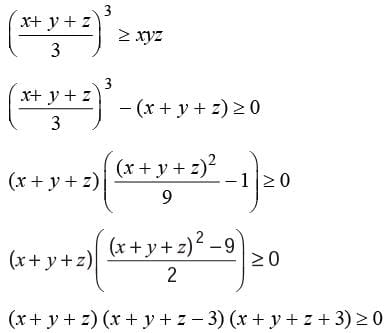∴ Sum of 3 natural numbers > 3.
∴ From general interpretation,
1 + 2  + 3 = 1 ⇒ 2 ⇒ 3
∴ Required set of natural numbers = (1, 2, 3).

QUESTION: 13

The square of a number is having 5 at its units place and 2 at its tenths place, then the least natural number having these properties are :

Solution:

∵ Last (unit) place digit = 5
∴ The least natural number whose perfect square is having 5, as its unit place digit will be equal to 5.
∴ 52 = 25

QUESTION: 14

The product 135 × 135 will be equal to :

Solution:

135 × 135
Trick :
135 × 135 = 25
Multiply the units place 5 and write the product, Add 1 to any one of the remaining digits at tenths place and write the product on tenths place.
13 × 14 = 182
∴ 135 × 135 = 18225

QUESTION: 15

Which of the following number is not a perfect squares ?

Solution:

∵ Perfect squares should have 1, 4, 9, 6 and 5 as their units place digit.
∴ 1282 is not a perfect square.

QUESTION: 16

26 + 34 × 17 ÷ 4 = 34, which of the two signs should be interchanged to get the desired result?

Solution:

Interchange of ×, ÷ will produce the desired result.

QUESTION: 17

Which is the least number divisible by 2, 3, 5 and 55 ?

Solution:

Least number which is divisible by 2, 3, 5 and 55
= LCM 2, 3, 5 and 55
= 66 × 5
= 330

QUESTION: 18

What is the square number just greater than 60, which can be expressed as a sum of two successive triangular numbers?

Solution:

60 < Sum of two triangular numbers = perfect square. The smallest number satisfying this condition is 64.

QUESTION: 19

What will be one’s place digit for 9201?

Solution:

91 = 9
92 = 81
93 = 243 × 3 = 729
94 = 6561
95 = 59049
∴ 94n = 6561
∴ 9200 has 1 as its units place digit.
∴ 9201 has 9 as its units place digit.

QUESTION: 20

What is the value of P if P, Q, R are replaced by digits from 1 to 9? PQ × QP = RQPR.

Solution: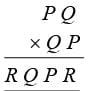P = 8, Q = 7, R = 6
∴ P = 8Use Code STAYHOME200 and get INR 200 additional OFF Use Coupon Code